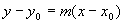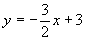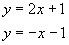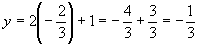Linear Functions and Straight Lines

Linear functions describe straight lines and have the general formThe number m is called the slope and determines the tilt of the line, while b is called the y-intercept, the point on the y-axis where the line crosses at x = 0. This form of a linear equation is often called slope-intercept form.   A typical graph of one of these functions looks like this:The slope is calculated from knowing any two distinct points on the line, (x1, y1) and (x2, y2)If the points are distinct, then the x coordinates cannot be the same and so zeros in the denominator are avoided.

The y-intercept can be calculated from any point if the slope is known. Since for any point (x1, y1)we must then haveGiven any two distinct points on the line, (x0, y0) and (x, y), we can set up two versions of the equation for the lineSubtracting, we getThis is the point-slope form.

Find the equations in slope-intercept and point-slope form for the line that passes through the pointsSolution

First calculate the slope:Then take one of the points and calculate the intercept:As a check, let’s do the same calculation with the other point:We got the same result with both points, a good indication that the calculations are correct. The equation of the line in slope-intercept form isand the equation in point-slope form isExample - Y-Intercept From Point-Slope Form

Given the following equation of a line in point-slope form:Find the y-intercept.

Solution

There are two ways to do this problem. The first is to multiply out the right-hand side of the equation and subtract 1 from both sides:So we now know the intercept is –7. The other way to do this problem is to use the intercept formula:We arrived at the same answer.

Example - Intercepts and Slope-Intercept Form

The y-intercept of a line is 3 and the x-intercept is 2. What is the equation of the line in slope-intercept form?

Solution

The y-intercept of a line is the y-coordinate where x = 0. Therefore, if the y-intercept is 3, the point on the line is (0, 3). In similar fashion, the x-intercept is the point on the x-axis where y = 0. Thus, if the x-intercept is 2, the point is (2, 0). With this information, we can apply the methods of the first example. First, calculate the slope:Now, we don’t have to calculate the y-intercept because it was given as 3! The equation of this line is:Example - Crossing Lines

At what point do the following two lines cross?Solution

The lines cross at a single point, so the lines must have the same x and y coordinates at that point.Now that we have the x-coordinate, we can use either equation to find the y-coordinate:Now we have both coordinates, so the answer is:Example - Perpendicular Lines

Find the line perpendicular tothat crosses this given line at (1, 17/6).

Solution

This example requires a formula for perpendicular lines, whose slopes are related bySo for our second (perpendicular) line, the slope isWe know that the point (1, 17/6) belongs to both lines, so we might as well use it. Plugging into the formula for the y-intercept,Thus the equation for the perpendicular line isExample - Parallel Lines

Find the line parallel to the linewhich passes through the point (-5, 1).

Solution

Parallel lines have the same slope, so we already know that m = 2. Plugging in to get the intercept yieldsSo the final line isback to examples